# Free Ncert Class 6 Math Mensuration Exercise 10.1

Mensuration Class 6 Ex. 10.1

Mensuration, Ncert Class 6, Chapter 10, Exercise 10.1 Math Free Solution.

Exercise 10.1

Question 1 :- Find perimeters of each of the following figures:

(a)(b)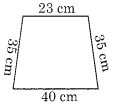(c)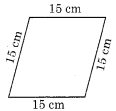(d)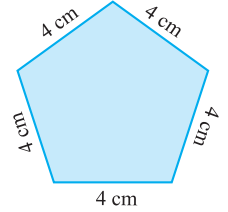(e)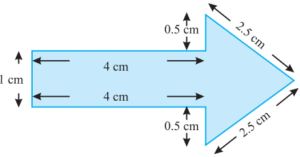(f)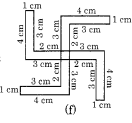Solution 1:-

(a) The perimeter = Sum of all the sides.
Required perimeter = 4 cm + 2 cm + 1 cm + 5 cm = 12 cm

(b) The perimeter = Sum of all the sides.
Required perimeter = 40 cm + 35 cm + 23 cm + 35 cm = 133 cm or 1.33 m

(c) The perimeter = Sum of all the sides.
Required perimeter = 15 cm + 15 cm + 15 cm + 15 cm  = 60 cm

(d)  The perimeter = Sum of all the sides.
Required perimeter = 4 cm + 4 cm + 4 cm + 4 cm + 4 cm  = 20 cm

(e) The perimeter = Sum of all the sides.
Required perimeter = 4 cm + 0.5 cm + 2.5 cm + 2.5 cm + 0.5 cm + 4 cm + 1 cm = 15 cm

(f) The perimeter = Sum of all the sides.
Required perimeter = 4 cm + 1 cm + 3 cm + 2 cm + 3 cm + 4 cm + 1 cm + 3 cm + 2 cm + 3 cm + 4 cm + 1 cm + 3 cm + 2 cm + 3 cm + 4 cm + 1 cm + 3 cm + 2 cm + 3 cm = 52 cm

Question 2 :- The lid of a rectangular-box of sides 40cm by 10cm is sealed all round with tapes. What is the length of the tapes required ?

Solution 2:-
The total length of the tape, which is required = the perimeter of the rectangle
= 2 x [40 + 10]
= 2 x 50 = 100 cm or 1 m.

Question 3 :- A table-top measures 2m 25cm by 1m 50cm. What is the perimeter of the table-top?

Solution 3:-
The length of table-top = 2m 25cm
The breadth of table-top = 1m 50cm
So,  Perimeter of the table top = perimeter of the rectangular = 2 [length + breadth]
= 2 [2m 25cm + 1m 50cm]
= 2 x (3m 75cm)
= 2 x (300 + 75) cm
= 2 x 375cm
= 750 cm
= 7 m 50 cm
= 7.5 m

Question 4 :- What is the length of the wooden-strip required; to frame a photograph of length and breadth 32cm and 21cm respectively ?

Solution 4:-
The length of the strip = 32cm
The breadth of the strip = 21cm
So, by the Formula of Perimeter of rectangle = 2 [length + breadth]
= 2 x [32 cm + 21 cm]
= 2 x (53)cm = 106 cm
So, the required length of the wooden-strip to frame = 106 cm or 1 m 6 cm.

Question 5 :- A rectangular piece-of-land measures 0.7km by 0.5km. Each-side is to be fenced with 4 rows of wire. What is the length-of-the-wire needed?

Solution 5:-
The length of the rectangular piece of land = 0.7 km = 0.7 x 1000 m = 700 m
The breadth of the rectangular piece of land = 0.5 km = 0.5 x 1000 m = 500 m
So, Perimeter of the rectangular land = 2 [length + breadth]
= 2 x [700 m + 500 m]
= 2400 m.
Hence, the length of the wire needed in 4 rounds of the land = 4 x 2400 = 9600 m = 9.6 km.

Question 6 :- Find the perimeter of each of the following shape:
(a) A triangle of sides 3cm, 4cm and 5cm;
(b) An equilateral-triangle of side 9 cm;
(c) An isosceles-triangle with equal-sides 8cm each and third side 6cm;

Solution 6:-
(a) We know, the perimeter of the triangles = The sum of all sides of the triangle;
So, the perimeter of the triangle = 3cm + 4cm + 5cm = 12cm.

(b) We know, the perimeter of the triangle = the sum of all the sides of the triangle
So, the perimeter of equilateral triangle = (9 + 9 + 9) cm = 27 cm.

(c) Perimeter of the given isosceles triangle = Sum of all the sides of the triangle
So, the perimeter of isosceles triangle = (8 + 8 + 6) cm = 22 cm.

Question 7 :- Find the perimeter of a triangle with sides measuring 10cm, 14cm and 15cm.

Solution 7:-
Perimeter of a triangle = Sum of all the sides of the triangle
= 10cm + 14cm + 15cm
= 39cm.

Question 8 :- Find the perimeter of a regular hexagon with each side measuring 8m.

Solution 8:-
Perimeter of a regular hexagon = 6 x side = 6 x 8 m = 48m.

Question 9 :- Find the side of the square whose perimeter is 20m.

Solution 9:-
The perimeter of a square = 4 x side
20 = 4 x side
Now, side = 20m/4 = 5 m.

Question 10 :-The perimeter of a regular-pentagon is 100cm. How long is its each side?

Solution 10:-

The perimeter of the regular pentagon = 100 cm
Number of sides in regular pentagon = 5
So, the perimeter of regular pentagon = 5 x sides
100 cm = 5 x side
side = 100/5 = 20cm.

Question 11 :- A piece of string is 30cm long. What will be the length of each-side; if the string is used to form:
(a) a square?
(b) an equilateral triangle?
(c) a regular hexagon?

Solution 11:-
(a) Length of the string = 30cm
The number of equal-sides in a square = 4;
So, the perimeter of the square = 4 x side
30 = 4 x side
side = 30/4 = 7.5cm.

(b) Length of string = 30 cm
Number of equal sides in equilateral triangle = 3
So, the perimeter of equilateral triangle = 3 x side;
30 = 3 x side;
side = 30/3 = 10cm.

(c) Length of string = 30 cm
The number of equal-sides in regular-hexagon = 6;
So, the perimeter of regular hexagon = 6 x side;
30 = 6 x side;
side = 30/6 = 5cm.

Question 12 :- Two-side of a triangle are 12cm and 14cm. The perimeter of the triangle is 36cm. What is its third side?

Solution 12:-
Perimeter of the triangle = 36cm.
Length of two of its sides are 12 cm and 14 cm.
So, the perimeter of triangle = sum of all the sides
36 = 12 + 14 + side
36 = 26 + side
side = 36 – 26 = 10cm

Hence the third side of triangle is 10cm.

Question 13 :- Find the cost of fencing a square park of side 250m at the rate of ₹20 per metre.

Solution 13:-
The length of the side of a square = 250m;
Since, the perimeter of the square = 4 x side
So, perimeter of the square =  4 x 250m = 1000 m
Since, rate of fencing = ₹20 per m.
So, the cost of fencing = ₹20 x 1000 = ₹20,000.

Hence, the total cost of fancing a square park is 20,000 rupees.

Question 14 :-Find the cost of fencing a rectangular-park of length 175m and breadth 125m at the rate of  ₹12(rupees) per metre.

Solution 14:-
The length of the rectangular-park = 175m;
The breadth of the rectangular-park = 125m;
So, Perimeter of the rectangular park = 2 [length + breadth]
= 2 x [175m + 125m]
= 2 x 300m = 600m

Rate of fencing = ₹12 per metre
The cost of fencing a rectangular park = ₹12 x 600 = ₹7200.

Hence, the total cost of fancing a rectangular park is 7,200 rupees.

Question 15 :- Sweety runs around a square-park of side 75m. Bulbul runs around a rectangular-park with length 60m and breadth 45m. Who covers less distance?

Solution 15:-
The side of the square park = 75m;
So, the perimeter of square park = 4 x side
= 4 x 75 m = 300m

Perimeter of the rectangular park = 2 [length + breadth]
= 2 x [60m + 45m]
= 2 x 105 m = 210m.
Since 210m < 300m.
So, Bulbul covers less distance.

Question 16 :- What is the perimeter of each of the following figures? What do you infer from the answers?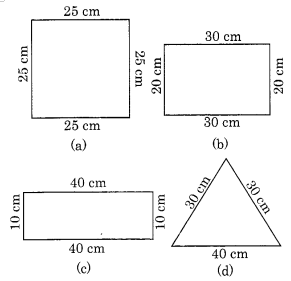Solution 16:-  Perimeter = sum of all the sides.

(a) Perimeter of the square = 25 cm + 25 cm + 25 cm + 25 cm
= 4 x 25 cm = 100cm.

(b) Perimeter of the rectangle = 30 cm + 20 cm + 30 cm + 20 cm
= 2 [30 cm + 20 cm] = 2 x 50 cm = 100cm.

(c) Perimeter of the rectangle = 40 cm + 10 cm + 40 cm + 10 cm
= 2 [40 cm + 10 cm] = 2 x 50 cm = 100 cm

(d) Perimeter of the triangle =  30 cm + 30 cm + 40 cm = 100 cm

From the above answers, we conclude that different figures may have equal perimeters.

Question 17 :- Avneet buys 9-square paving slabs, each with a side of 1/2 m. He lays them in the form of asquare.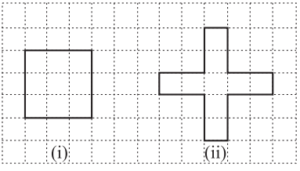(a) What is the perimeter of his arrangement [in figure. (i)]?
(b) Shari does not like his arrangement. She gets him to lay them out like a cross. What is the perimeter of her arrangement [Fig. (ii)]?
(c) Which has greater perimeter?
(d) Avneet wonders, if there is a way of getting an even greater-perimeter. Can you find a way of doing this? (The paving slabs must meet along complete edges, i.e., they cannot be broken).

Solution 17:-
(a) Perimeter of square-arrangement = 6m.

(b) Perimeter of cross-arrangement = 10m.

(c) Since ,10 m > 6 m
So, the cross-arrangement has greater perimeter.

(d) Total number of tiles = 9
So, We have the following arrangement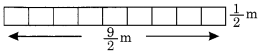The above arrangement will also have the greater perimeter.